# 0708-1300/Class notes for Tuesday, February 26

Announcements go here

## A Homology Theory is a Monster

Bredon's Plan of Attack: State all, apply all, prove all.

Our Route: Axiom by axiom - state, apply, prove. Thus everything we will do will be, or should be, labeled either "State" or "Prove" or "Apply".

## Typed Notes

The notes below are by the students and for the students. Hopefully they are useful, but they come with no guarantee of any kind.

### First Hour

Recall we had defined for a chain complex the associated homology groups: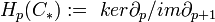$H_p(C_*) :=\ ker\partial_p/im\partial_{p+1}$

From this we get the pth homology for a topological space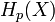$H_p(X)$

We have previously shown that

1)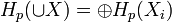$H_p(\cup X) = \oplus H_p(X_i)$ for disjoint unions of spaces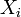$X_i$

2)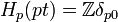$H_p(pt) = \mathbb{Z}\delta_{p0}$

3)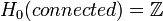$H_0(connected) = \mathbb{Z}$

4)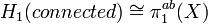$H_1(connected) \cong \pi_1^{ab}(X)$ via the map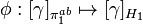$\phi:[\gamma]_{\pi_1^{ab}}\mapsto[\gamma]_{H_1}$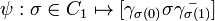$\psi:\sigma\in C_1\mapsto[\gamma_{\sigma(0)}\sigma\bar{\gamma_{\sigma(1)}}]$ where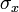$\sigma_x$ is a path connecting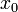$x_0$ to x.

We need to check the maps are in fact inverses of each other.

Lets consider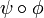$\psi\circ\phi$. We start with a closed path starting at$x_0$ (thought of as in the fundamental group).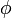$\phi$ means we now think of it as a simplex in X with a point at$x_0$.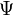$\Psi$ now takes this to the path that parks at$x_0$ for a third of the time, goes around the loop and then parks for the remaining third of the time. Clearly this is homotopic this composition is homotopic to the identity.

We now consider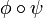$\phi\circ\psi$. Start with just a path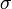$\sigma$. Then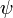$\psi$ makes a loop adding two paths from the$x_0$ to the start and finish of$\sigma$ forming a triangular like closed loop. We think of this loop as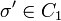$\sigma'\in C_1$

Now, we start from c being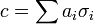$c = \sum a_i\sigma_i$ with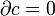$\partial c = 0$. So get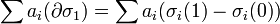$\sum a_i(\partial \sigma_1) = \sum a_i(\sigma_i(1)-\sigma_i(0))$

So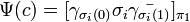$\Psi(c) = [\gamma_{\sigma_i(0)}\sigma_i\bar{\gamma_{\sigma_i(1)}}]_{\pi_1}$ which maps to, under$\phi$,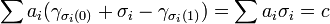$\sum a_i(\gamma_{\sigma_i(0)} + \sigma_i - \gamma_{\sigma_i(1)}) = \sum a_i\sigma_i = c$ ( in the homology gamma's cancel as$\partial c = 0$)

Axiomized Homology

We now will move to an approach where we prove that our defined homology satisfies a series of established homology axioms that will allow us to apply the machinery of general homology to our specific "singular" homology defined via simplices.

Axiom 0) Homology if a function

Definition The "category of chain complexes" is a category whose objects are chain complexes (of abelian groups) and morphisms which is a homomorphism between each abelian group in one chain and the corresponding group in the other chain such that the resulting diagram commutes. I.e,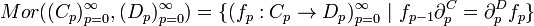$Mor((C_p)_{p=0}^{\infty}, (D_p)^{\infty}_{p=0}) = \{(f_p:C_p\rightarrow D_p)_{p=0}^{\infty}\ |\ f_{p-1}\partial_p^C=\partial_p^D f_p\}$

Now, in our case, the chain complexes do in fact commute because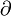$\partial$ is defined by pre-composition but f is defined by post-composition. Hence, associativity of composition yields commutativity.

Claim

Homology of chain complexes is a functor in the natural way. That is, if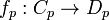$f_p:C_p\rightarrow D_p$ for each p induces the functor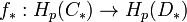$f_*:H_p(C_*)\rightarrow H_p(D_*)$

The proof is by "diagram chasing". Well, let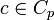$c\in C_p$,$\partial c =0$.

Let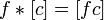$f*[c] = [fc]$. Now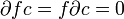$\partial fc = f\partial c = 0$. Furthermore, suppose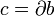$c = \partial b$. Then,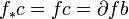$f_*c = fc = \partial fb$ so therefore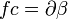$fc = \partial\beta$ some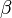$\beta$. This shows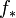$f_*$ is well defined.

Thus, for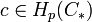$c\in H_p(C_*)$ get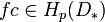$fc\in H_p(D_*)$ via the well defined functor$f_*$.